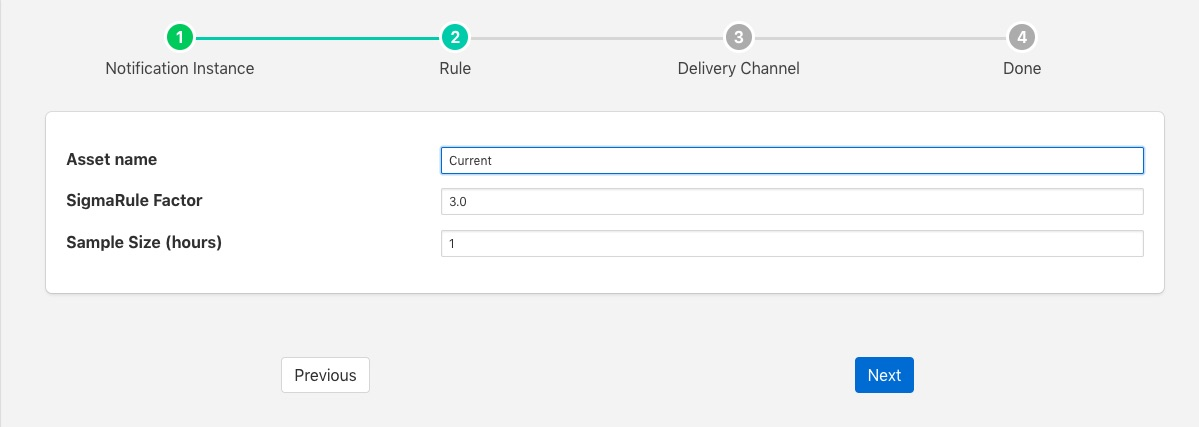# Simple-Sigma Rule¶

The foglamp-rule-simple-sigma is a notification rule plugin that uses the principle of normal distribution to trigger a notification if a value is found to be an outlier, a value that is outside of the normal distribution. The normal distribution is discovered by taking the mean of all the values over time and calculating the standard deviation, or sigma, from that mean. Until the rule has built up a reasonable sample of data on which to calculate the mean and standard deviation the rule will not trigger. This reasonable sample is defined as a time period, in hours, for which the rule will simply sample the data to determine the mean and sigma values. During this period the rule will not trigger. The default time period for this is 1 hour, however it may be overridden.

Once a mean and standard deviation have been determined the rule will mode into a mode in which it will trigger. Whilst in triggering mode the rule will still refine the mean and standard deviation values. If a value is found in trigger mode that is more than a certain number of standard deviations from the mean, then the rule will trigger. The number of standard deviations is the sigma factor and defaults to 3.0, however the user can configure this to be more or less than 3.0.

To use the Simple-Sigma plugin create your notification rule as normal, when selecting the rule to use select the Simple-Sigma rule and click on next. You will be presented with a dialog as belowConfigure the Simple-Sigma rule

• Asset name: The asset name to monitor with the rule

• Sigma Factor: The factor to use for determining range, a factor of 3.0 will trigger when a value is more the 3.0 * Sigma from the current mean

• Sample Size: The number of hours to build a mean and standard deviation before the rule will trigger.